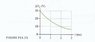# Resistance from a V-time graph

max1205

## Homework Statement

A 50 uF(microfarad) capacitor that had been charged to 30 V is discharged through a resistor. Figure 31.73 (attachment) shows the capacitor voltage as a function of time. What is the value of the resistance?

## Homework Equations

Q = Qo e^(-t/RC), o = initial

Qo = CV, o = initial

I = Io e^(-t/RC), o = initial

## The Attempt at a Solution

Original V = 30, the final V at 3ms is approximately 7V. Thus the final charge is 0.233 (7/30) of the initial charge.

Q = Qo e^(-t/RC)
ln (7/30) = -3/(R)(50 uF)

R = 0.041 ohms

The right answer is 36.4 ohms.

Any suggestions?

#### Attachments

•figure 31.73.jpg
7.7 KB · Views: 1,753

naele
$$R = \frac {-t}{\ln\frac{V}{Vo}C}$$. However that gives me 41.22 ohm so maybe there's a typo in the problem?

edit2: actually you didn't solve for 1/R like I first thought, you're just off by 3 orders of magnitude because you probably forgot to convert the milliseconds to seconds.

Last edited:
max1205
does anyone have an idea?

naele
Well you estimated the 7V at 3ms since apparently all you had to go by was that graph. If you plug in 36.4 ohms as the resistance into the equation you get something like 5.8V which makes sense. Kind of a lame question if they don't give exact values.

max1205
thanks!

JosieNutter
Don't estimate - you can see exactly what V is at 2ms. R is constant.

V = V_0 * e^(-t/RC)

Solve for R an input data from the graph where t=2ms.